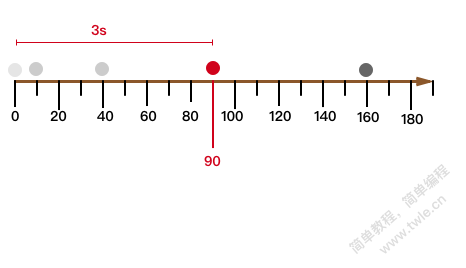Canvas 加速运动

加速运动运动

匀加速直线运动```v = v0 + a * t;
```

```v2 = v1 + a * t;
```

```(v2-v1)/t = a
```

匀加速直线运动的距离

```S + v0 * t + (a * t * t) / 2
```

1. 先看看运动 `1s` 后的速度，为 `v = v0 + a` ,应该能理解吧

比如小车的初始速度为 80，加速度为 20，那么 1 秒后的速度为 100

2. 第二结束的速度是多少呢？

经过第一秒的加速，第二秒开始时的速度 `v0+a`，那么它在第二秒结束的速度为

```v = (v0 + a) + a = v0 + 2*a;
```
3. 第三秒结束后的速度时多少呢？

经过前两秒的加速，第三秒开始的 `v0+a+a`，也就是 `v0+2*a`，那么它在第三秒结束时的速度为

```v = (v0 + 2*a) + a = v0 + 3*a;
```
4. 那么以此类推，第 t 秒结束时的速度为

```v = ((((v0 + a) + a)+a)+a)...
```

我们可以看到总共有 t 个 `a` ，也就是

```v = v0 + a * t
```
5. 然后我们就可以求出 t 秒内的平均运行速度为

```v' = (v0 + (v0+a*t))/ 2 = v0 + (a*t)/2
```
6. 那么就可以得到 t 秒内运行的距离为

```S = v' * t = (v0 + (a*t)/2) * t

= v0 * t + a*t*t/2
```

```S = v * t
```

匀加速(减速)直线运动动画

```<!DOCTYPE html>
<meta charset="utf-8">
<canvas id="canvas-1" width="400" height="200">
</canvas>
<script>
var c = document.getElementById('canvas-1');
var ctx = c.getContext('2d');
var rq = null;

var ball = {
x: 10,
y: 10,
vx: 5,
vy: 2,
color: 'blue',
draw: function() {
ctx.beginPath();
ctx.arc(this.x, this.y, this.radius, 0, Math.PI * 2, true);
ctx.closePath();
ctx.fillStyle = this.color;
ctx.fill();
}
};

function draw() {
ctx.clearRect(0,0, c.width, c.height);
ctx.strokeRect(0,0,c.width, c.height);
ball.draw();
ball.x += ball.vx;
ball.y += ball.vy;
ball.vy *= .99;
ball.vy += .25;

if (ball.y + ball.vy > c.height ||
ball.y + ball.vy < 0) {
ball.vy = -ball.vy;
}
if (ball.x + ball.vx > c.width ||
ball.x + ball.vx < 0) {
ball.vx = -ball.vx;
}

rq = window.requestAnimationFrame(draw);
}

var rq = window.requestAnimationFrame(draw);
ball.draw();
</script>
```

Canvas 基础教程

简单教程，简单编程 - IT 入门首选站